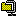## String encrypters with AES-256 support.

2012. 12. 10. 01:04RyeolStringEncrypter.zip

This is new string encrypters that is the upgrade of the previous string encrypters to support AES-256. Though PHP and Java are not available, you can download a COM dll (32/64 bit) and a C# class.

The string encrypters take two strings, a key and an initialization vector. To be used as an AES key, the key string is converted into UTF-8, and then is hashed by MD5 or SHA2-256.Similarly, the IV is hashed by MD5.The input string is converted into UTF-8, and then is encrypted using 128-bits or 256-bits AES in Cipher Block Chaining(CBC) with PKCS7 padding. The AES key size depends on the size of the hash for the key string. For example, if the hash is SHA2-256, 256-bits AES is used. Base64 is used for the encrypted binary.The following code is an example of using the string encrypter in C#. The StringEncrypter class is implemented in the StringEncrypter.cs file.

```/*
*  C#
*/
using Ryeol.Security.Cryptography;
...
const string key = "This is a secret key.";
const string iv = "This is an initialization vector.";

// Creates an instance.
StringEncrypter encrypter = new StringEncrypter(key, iv);

// Sets the key hash algorithm. MD5 and SHA2-256 are supported.
// In this case the hash size is 256 bits, AES-256 is used.
encrypter.KeyHashAlgorithm = StringEncrypterKeyHashAlgorithm.SHA2_256;

// Encrypts a string.
string encrypted = encrypter.Encrypt("Test sample");

// Decrypts the encrypted string.
string decrypted = encrypter.Decrypt(encrypted);
```

The following code shows how to use the string encrypter in ASP(VBScript). Since ASP doesn't support built-in AES algorithm, you need to install the RyeolStringEncrypter.dll file which is a COM dll based on XySSL 0.8.
(You also need to install the Visual C++ Redistributable for Visual Studio 2012.)
```'
'  ASP (VBScript)
'
Const conKey = "This is a secret key."
Const conIV = "This is an initialization vector."

' Creates an instance.
Set objEncrypter = Server.CreateObject("Ryeol.StringEncrypter")

objEncrypter.Key = conKey
objEncrypter.IV = conIV

' Sets the key hash algorithm. MD5 and SHA2-256 are supported.
' In this case the hash size is 256 bits, AES-256 is used.
objEncrypter.KeyHashAlgorithm = "SHA2-256"

' Encrypts a string.
strEncrypted = objEncrypter.Encrypt("Test sample")

' Decrypts the encrypted string.
strDecrypted = objEncrypter.Decrypt(strEncrypted)
```

Release Note

June 7, 2013
The C# StringEncrypter has changed to create a hash implementation by using the Create method to support Windows XP.

December 10, 2012
Initial release.
YOUR COMMENT IS THE CRITICAL SUCCESS FACTOR FOR THE QUALITY OF BLOG POST